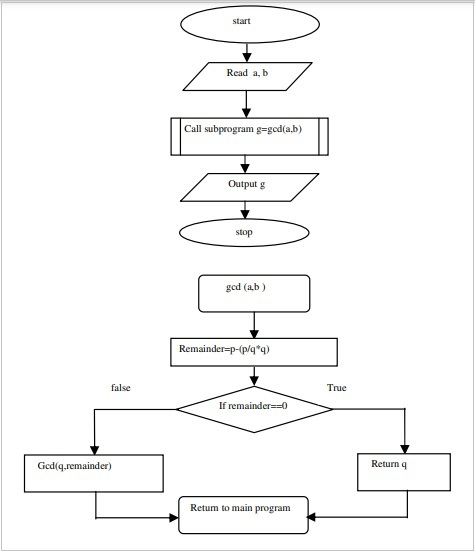# C program to find GCD of numbers using non-recursive function

## Problem

Find the greatest common divisor (GCD) for the given two numbers by using the non-recursive function.

## Solution

It is explained below how to find the greatest common divisor (GCD) for the given two numbers by using the non-recursive function.

## Algorithm

Refer an algorithm given below to find the greatest common divisor (GCD) for the given two numbers by using the non-recursive function.

Step 1 − Start

Step 2 − Read the integers a and b

Step 3 − Call the function G=GCD(a,b) step 6

Step 4 − Print G value

Step 5 − Stop

Step 6 − Called function: GCD(a,b)

a. Initialize the i=1, j, remainder
b. Remainder=i-(i/j*j)
c. Remainder=0 return j else goto step 4
d. GCD(G,remainder) return to main program

## Flowchart

Given below is a flowchart for an algorithm to find the greatest common divisor (GCD) for the given two numbers by using the non-recursive function.## Example

Following is the C program to find the greatest common divisor (GCD) for the given two numbers by using the non-recursive function

#include<stdio.h>
#include<conio.h>
#include<math.h>
int gcdnonR(int i,int j){
int rem;
rem=i-(i/j*j);
if(rem==0)
return j;
else
gcdnonR(j,rem);
}
void main(){
int a,b;
printf("enter the two numbers:");
scanf("%d%d",&a,&b);
printf("GCD of %d",gcdnonR(a,b));
getch();
}

## Output

When the above program is executed, it produces the following result −

enter the two numbers:10 30
GCD of 10